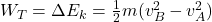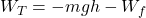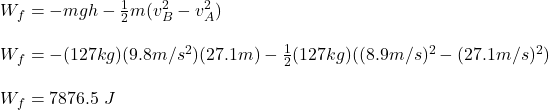## You are testing a new amusement park roller coaster with an empty car with mass 127 kg. One part of the track is a vertical loop with radius

Question

You are testing a new amusement park roller coaster with an empty car with mass 127 kg. One part of the track is a vertical loop with radius 11.8 m. At the bottom of the loop (point A) the car has speed 27.1 m/s, and at the top of the loop (point B) it has speed 8.9 m/s. As the car rolls from point A to point B, how much work is done by the friction

in progress 0
4 months 2021-09-05T10:22:46+00:00 1 Answers 2 views 0

Wf = 7876.5 J

Explanation:

To find the work done by the friction force you use the following formula, in which the total work is given by the change in the kinetic energy of the car:(1)

m: mass of the car = 127 kg

vB: speed at the top of the loop = 8.9 m/s

vA: speed at the bottom of the loop = 27.1 m/s

The total work is the contribution of the work done by the gravitational force and also the work done by the friction force, that is:(2)

g: gravitational acceleration = 9.8m/s^2

h: height of the car to the ground at point B = 2R = 2(11.8m) = 23.6 m

Then, you first equal the equations (1) and (2). Next, you replace the values of vB, vA, m, g and h, in order to obtain the work done by the friction force: